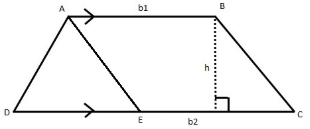# Perimeter of a Trapezoid Formula

## Perimeter of a Trapezoid Formula - Trapezoid Area Formula

A trapezium is a quadrilateral in which exactly one pair of opposite sides are parallel. Consider the following trapezium whose height and side lengths are known.The perimeter of the trapezium is simply the sum of the lengths of its sides.
$perimeter = AB + BC + CD + DA$

To find the area of the trapezium, we break it into a parallelogram and a triangle, as shown in the diagram.
$\begin{gathered} area\left( {trapezium\,ABCD} \right) = area\left( {{\text{triangle}}\,ADE} \right) + area\left( {{\text{parallelogram}}\,ABCE} \right) \\ = \frac{1}{2} \times DE \times h + EC \times h \\ \end{gathered}$
Since opposite sides of a parallelogram are equal, $EC = AB = {b_1}$
$DE = DC - EC = {b_2} - {b_1}$
$\Rightarrow area\left( {trapezium\,ABCD} \right) = \frac{h}{2} \times \left( {DE + 2EC} \right) = \frac{h}{2} \times \left( {{b_2} - {b_1} + 2{b_1}} \right) = \frac{h}{2}\left( {{b_1} + {b_2}} \right)$
Let’s look at an example to see how to use these formulas.

Question: Calculate the perimeter and the area of a trapezium of height 5 cm if its parallel sides are 4 cm and 10 cm and its non-parallel sides are 6 cm and 8 cm.
Solution:
$\begin{gathered} {\text{perimeter}} = 4 + 10 + 6 + 8 = 28\,cm \hfill \\ {\text{area}} = \frac{h}{2}\left( {{b_1} + {b_2}} \right) = \frac{5}{2} \times \left( {4 + 10} \right) = 35\,c{m^2} \hfill \\ \end{gathered}$
Why don’t you try solving the following problem now?

Question: A trapezium has an area of $20\,c{m^2}$. If its height is 4 cm and its non-parallel sides are 6 cm and 5 cm , then evaluate its perimeter.
Options:
(a) $21\,cm$
(b) $25\,cm$
(c) $27\,cm$
(d) none of these
${\text{area}} = \frac{h}{2}\left( {{b_1} + {b_2}} \right) \Rightarrow 20 = \frac{4}{2} \times \left( {{b_1} + {b_2}} \right) \Rightarrow {b_1} + {b_2} = 10\,cm$
${\text{perimeter}} = 6 + 5 + {b_1} + {b_2} = 11 + 10 = 21\,cm$